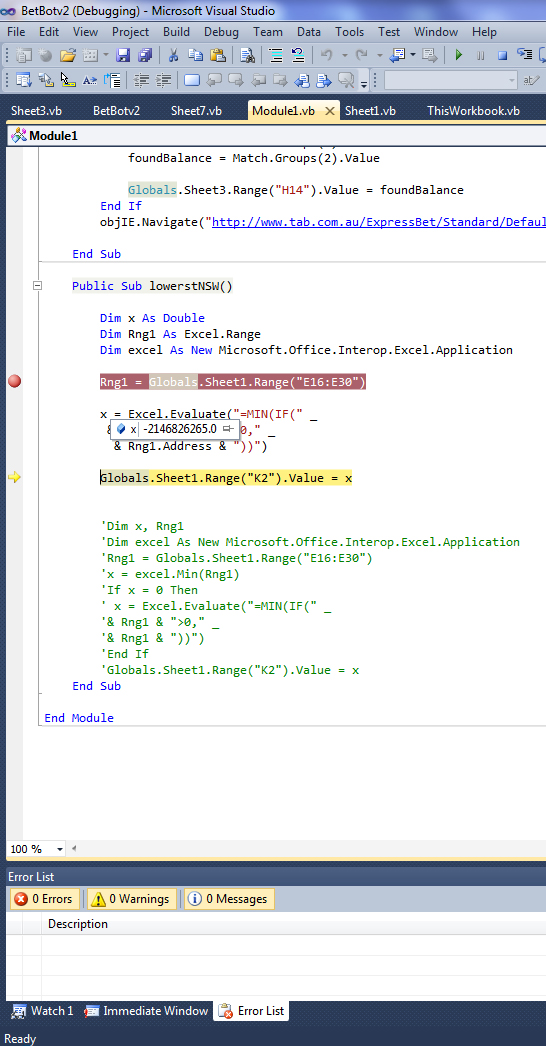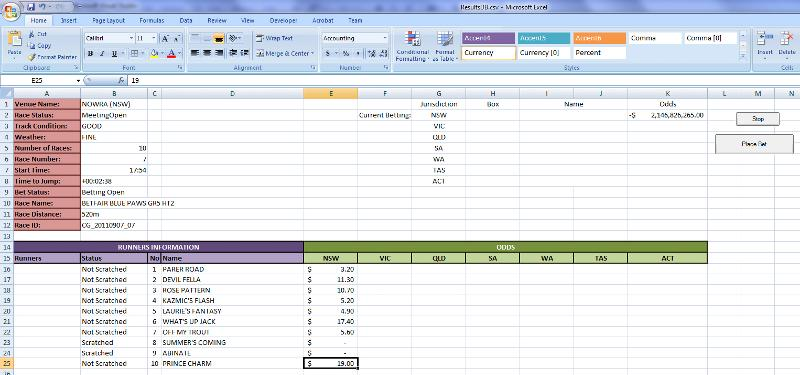# Excel VBA code to find the lowest number in range &gt;0

The code I got is erroring

First step
What I am trying to do is I have a range which I need to find the lowest value greater than 0

Second step
Then get the content of a range in that row and display in different cells
I haven't started step to still trying to resolve step 1

``````Public Sub lowerstNSW()

Dim x, Rng1
Dim excel As New Microsoft.Office.Interop.Excel.Application
Rng1 = Globals.Sheet1.Range("E16:E30")
x = excel.Min(Rng1)
If x = 0 Then
x = excel.Evaluate("=MIN(IF(" _
End If
Globals.Sheet1.Range("K2").Value = x
End Sub
``````
VB ScriptMicrosoft ExcelLast Comment
JasonsnailcatTry this:
``````Public Sub lowerstNSW()

Dim x As Integer
Dim Rng1 As Range

Set Rng1 = Range("E16:E30")

x = excel.Evaluate("=MIN(IF(" _

Sheet1.Range("K2").Value = x

End Sub
``````snailcatI am assuming with the prior code that x is an integer.
Will you always have a positive number greater than 0 in the range?

If not what would you want the value of x to be?  (if your values were -5, -4, 0 then what should happen?)JasonYes will always be positivesnailcatJasonit returns a huge negative number

the lowest number in the range is 4.90 other than 2 zerossnailcatsnailcatHere is the code that will return non-integer numbers :
``````Public Sub lowerstNSW()

Dim x As Double

Dim Rng1 As Range

Set Rng1 = Range("E16:E30")

x = Excel.Evaluate("=MIN(IF(" _

Sheet1.Range("K2").Value = x

End Sub
``````JasonCant post the spreadsheet you would not be able to use it.  It requires xml auth login to tab website

Did the change still comes up with huge negative number.andrewssd3I may be missing something here, but why not just put the formula into cell K2 in one step like this:

``````Public Sub lowerstNSW()

Dim x As Double

Dim Rng1 As Range

Set Rng1 = Range("E16:E30")

Range("K2").FormulaArray = "=MIN(IF(" & _

End Sub
``````Jasonandrew thanks heaps Great Job

now can you help with getting the name in I2 and the Box in H2

Based in the K2 results

The Range to search is C16: D30andrewssd3I'm not quite clear exactly what you want here - I am assuming it is some sort of lookup from the row where the min value occurs.

I suggest you raise this as a new question with a proper explanation and close this one (awarding points as necessary!), as there should really only be one question per thread.JasonHi andrew

I am happy to do that will you be available to assist after all it was all stated in the initial posting

If you look at the spreadsheet img you can clearly see what I am askingsnailcatDo you want appropriate value in Column D to go to I2 and Column C to H2?andrewssd3THIS SOLUTION IS ONLY AVAILABLE TO MEMBERS.
View this solution by signing up for a free trial.
Members can start a 7-Day free trial and enjoy unlimited access to the platform.andrewssd3Sorry - meant to say that this does not address what would happen if the minimum value occurred 2 or more times in the reference table - this would just find the first one.Jasonandrewssd3Forgot you were in VS - the enum for xlR1C1 is called xlReferenceStyle - so try Excel.xlReferenceStyle.xlR1C1JasonGreat Work Andrew

Thanks for your help works a dreamMicrosoft Excel

Microsoft Excel topics include formulas, formatting, VBA macros and user-defined functions, and everything else related to the spreadsheet user interface, including error messages.

144K
Questions
--
Followers
--
Top Experts
Get a personalized solution from industry experts

TRUSTED BY